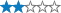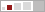More
<

## Random by Randolph

A cache by dgauss Message this owner
Hidden : 04/14/2009
Difficulty:Terrain:Size:(small)

#### Watch

How Geocaching WorksPlease note Use of geocaching.com services is subject to the terms and conditions in our disclaimer.

## Geocache Description:

NOT at the listed coordinates. You get to select the coordinates.

We need some numbers:

A) Pick a number between 12 and 5, the very first number that comes to mind. Call it A.

B,C) Roll a die getting a number between 1 and 6. Multiply 142857 by that number. Next add together the digits in that product. You should get a two-digit number. Call it BC.

D) Cut a deck of cards. Consider the denomination of the card cut. Use a 1 for an ace, 2 for a deuce, ..., 13 for a king. Now multiply the denomination (1..13) by 9. Add the digits in the result. If that sum is a single digit, stop. Otherwise, add the digits in that sum to get a second sum. Etc. Stop when you get a single digit number. Call it D.

E,F,G) What's your age? Multiply it by 5 and add 66. Now double the result and subtract 11. You should have a three-digit result. Call it EFG.

H,I) Pick a highway, any two-digit, north-south highway passing through Randolph. Call it HI.

J,K) Pick an unlucky number between 10 and 20. Call it JK.

L) Let LL = JK + KJ.

M) Let M = L/2.

O) Divide the difference between EF and FE by 9. Let O be the remainder.

P) Let n = (A+B+C+D) - (H+I+J+K+L+M+O). Think of a country that begins with the nth letter of the alphabet ('A' if n = 1, 'B' if n = 2, ..., 'Z' if n = 26.) Now think of an animal that begins with the last letter in the name of your country. Let P = the number of letters in the name of your animal.

The cache is at

### N LL° KJ.GPD, W ODM° HI.ABC

Check your exact answer here. If it matches go get it. If it doesn't, check your arithmetic or go get a friend.

Winter Friendliness: Should tolerate a foot or two of snow.